# Tamil Nadu Board Samacheer Kalvi solutions for Class 4th Mathematics Term 2 Answers Guide chapter 6 - Fraction [Latest edition]

#### Chapters## Chapter 6: Fraction

Exercise 6.1Exercise 6.2Exercise 6.3Exercise 6.4Exercise 6.5Exercise 6.6
Exercise 6.1 [Page 37]

### Tamil Nadu Board Samacheer Kalvi solutions for Class 4th Mathematics Term 2 Answers Guide Chapter 6 FractionExercise 6.1 [Page 37]

Exercise 6.1 | Q 1. (i) | Page 37

Observe the following picture and write how many equal parts are they divided into?Exercise 6.1 | Q 1. (ii) | Page 37

Observe the following picture and write how many equal parts are they divided into?Exercise 6.1 | Q 1. (iii) | Page 37

Observe the following picture and write how many equal parts are they divided into?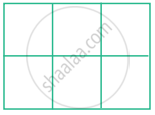Exercise 6.1 | Q 1. (iv) | Page 37

Observe the following picture and write how many equal parts are they divided into?Exercise 6.1 | Q 2 | Page 37

Draw square and circle. Make it into four equal parts.

Exercise 6.1 | Q 3 | Page 37

Draw a rectangle and divide it into eight equal parts

Exercise 6.2 [Page 39]

### Tamil Nadu Board Samacheer Kalvi solutions for Class 4th Mathematics Term 2 Answers Guide Chapter 6 FractionExercise 6.2 [Page 39]

Exercise 6.2 | Q 1. (i) | Page 39

Write the fractional part for the picture that is circled.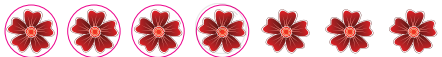Exercise 6.2 | Q 1. (ii) | Page 39

Write the fractional part for the picture that is circled.Exercise 6.2 | Q 1. (iii) | Page 39

Write the fractional part for the picture that is circled.Exercise 6.2 | Q 2 | Page 39

Match the following:

 Column A Column B3/72/5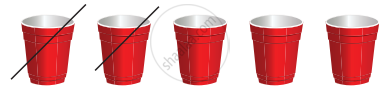4/9
Exercise 6.2 | Q 3 | Page 39

Mark the given pictures with the fractions given below.

 1 3/82 2/7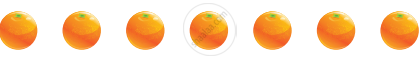3 5/9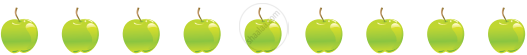Exercise 6.3 [Page 42]

### Tamil Nadu Board Samacheer Kalvi solutions for Class 4th Mathematics Term 2 Answers Guide Chapter 6 FractionExercise 6.3 [Page 42]

Exercise 6.3 | Q I. 1. | Page 42

Write the numerator and denominator for the following numbers.

3/7

Exercise 6.3 | Q I. 2. | Page 42

Write the numerator and denominator for the following numbers.

4/6

Exercise 6.3 | Q I. 3. | Page 42

Write the numerator and denominator for the following numbers.

5/10

Exercise 6.3 | Q I. 4. | Page 42

Write the numerator and denominator for the following numbers.

1/3

Exercise 6.3 | Q II. 1. | Page 42

Shade the part according to the number and write the numerator and denominator.

 2/8Numerator/Denominator
Exercise 6.3 | Q II. 2. | Page 42

Shade the part according to the number and write the numerator and denominator.

 3/4Numerator/Denominator
Exercise 6.3 | Q II. 3. | Page 42

Shade the part according to the number and write the numerator and denominator.

 5/10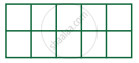Numerator/Denominator
Exercise 6.3 | Q II. 4. | Page 42

Shade the part according to the number and write the numerator and denominator.

 1/4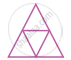Numerator/Denominator
Exercise 6.3 | Q II. 5. | Page 42

Shade the part according to the number and write the numerator and denominator.

 3/8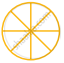Numerator/Denominator
Exercise 6.4 [Page 44]

### Tamil Nadu Board Samacheer Kalvi solutions for Class 4th Mathematics Term 2 Answers Guide Chapter 6 FractionExercise 6.4 [Page 44]

Exercise 6.4 | Q I. | Page 44

Colour or shade half portion of the following pictures: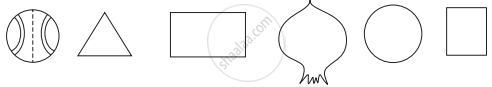Exercise 6.4 | Q II. | Page 44

Tick the picture that represent semi portion.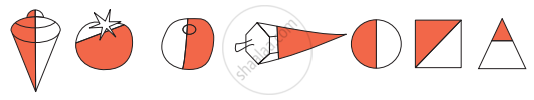Exercise 6.4 | Q III. (i) | Page 44

Write appropriately which represent half, quarter, three fourth.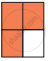Exercise 6.4 | Q III. (ii) | Page 44

Write appropriately which represent half, quarter, three fourth.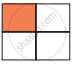Exercise 6.4 | Q III. (iii) | Page 44

Write appropriately which represent half, quarter, three fourth.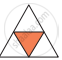Exercise 6.4 | Q III. (iv) | Page 44

Write appropriately which represent half, quarter, three fourth.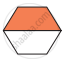Exercise 6.4 | Q III. (v) | Page 44

Write appropriately which represent half, quarter, three fourth.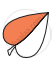Exercise 6.4 | Q III. (vi) | Page 44

Write appropriately which represent half, quarter, three fourth.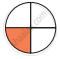Exercise 6.4 | Q III. (vii) | Page 44

Write appropriately which represent half, quarter, three fourth.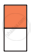Exercise 6.5 [Pages 46 - 47]

### Tamil Nadu Board Samacheer Kalvi solutions for Class 4th Mathematics Term 2 Answers Guide Chapter 6 FractionExercise 6.5 [Pages 46 - 47]

Exercise 6.5 | Q I. (i) | Page 46

Write the fraction for the shaded portions of the given figure.Exercise 6.5 | Q I. (ii) | Page 46

Write the fraction for the shaded portions of the given figure.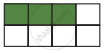Exercise 6.5 | Q I. (iii) | Page 46

Write the fraction for the shaded portions of the given figure.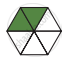Exercise 6.5 | Q I. (iv) | Page 46

Write the fraction for the shaded portions of the given figure.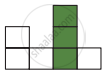Exercise 6.5 | Q I. (v) | Page 46

Write the fraction for the shaded portions of the given figure.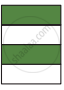Exercise 6.5 | Q II. | Page 47

Shade the figure according to the fraction

 1 3/42 5/10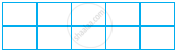3 1/8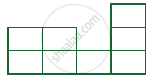4 2/6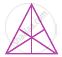Exercise 6.6 [Page 50]

### Tamil Nadu Board Samacheer Kalvi solutions for Class 4th Mathematics Term 2 Answers Guide Chapter 6 FractionExercise 6.6 [Page 50]

Exercise 6.6 | Q I. 1. | Page 50

Circle the greater fraction

1/3, 2/3

Exercise 6.6 | Q I. 2. | Page 50

Circle the greater fraction

3/4, 1/4

Exercise 6.6 | Q I. 3. | Page 50

Circle the greater fraction

2/5, 4/5

Exercise 6.6 | Q I. 4. | Page 50

Circle the greater fraction

6/8, 3/8

Exercise 6.6 | Q I. 5. | Page 50

Circle the greater fraction

4/10, 3/10

Exercise 6.6 | Q I. 6. | Page 50

Circle the greater fraction

2/9, 7/9

Exercise 6.6 | Q II. 1. | Page 50

Tick the smaller fraction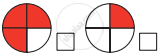Exercise 6.6 | Q II. 2. | Page 50

Tick the smaller fraction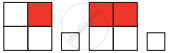Exercise 6.6 | Q II. 3. | Page 50

Tick the smaller fraction## Chapter 6: Fraction

Exercise 6.1Exercise 6.2Exercise 6.3Exercise 6.4Exercise 6.5Exercise 6.6## Tamil Nadu Board Samacheer Kalvi solutions for Class 4th Mathematics Term 2 Answers Guide chapter 6 - Fraction

Tamil Nadu Board Samacheer Kalvi solutions for Class 4th Mathematics Term 2 Answers Guide chapter 6 (Fraction) include all questions with solution and detail explanation. This will clear students doubts about any question and improve application skills while preparing for board exams. The detailed, step-by-step solutions will help you understand the concepts better and clear your confusions, if any. Shaalaa.com has the Tamil Nadu Board of Secondary Education Class 4th Mathematics Term 2 Answers Guide solutions in a manner that help students grasp basic concepts better and faster.

Further, we at Shaalaa.com provide such solutions so that students can prepare for written exams. Tamil Nadu Board Samacheer Kalvi textbook solutions can be a core help for self-study and acts as a perfect self-help guidance for students.

Concepts covered in Class 4th Mathematics Term 2 Answers Guide chapter 6 Fraction are Concept of Fractions.

Using Tamil Nadu Board Samacheer Kalvi Class 4th solutions Fraction exercise by students are an easy way to prepare for the exams, as they involve solutions arranged chapter-wise also page wise. The questions involved in Tamil Nadu Board Samacheer Kalvi Solutions are important questions that can be asked in the final exam. Maximum students of Tamil Nadu Board of Secondary Education Class 4th prefer Tamil Nadu Board Samacheer Kalvi Textbook Solutions to score more in exam.

Get the free view of chapter 6 Fraction Class 4th extra questions for Class 4th Mathematics Term 2 Answers Guide and can use Shaalaa.com to keep it handy for your exam preparation